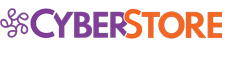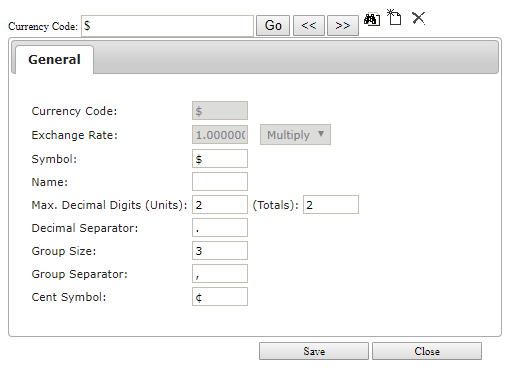﻿ Formatting Prices as CurrencyCyberStore Documentation
Formatting Prices as Currency
 User's Guide > Business Logic > Pricing > Formatting Prices as Currency

In CyberStore, Currency defines a format that is able to be applied to numbers that represent monetary values. Currency formats are setup in the Setup > Currency screen in the Management Console.Each Currency format applies to a single Currency Code as setup in SYSPRO, and each Customer has a single Currency Code associated with their SYSPRO Customer setup.

CyberStore assigns the Currency Format based on the Account logged in, and the current Customer that they are actively associated with at that time. This remains the format that will be used until the shopper changes their Account to a differenct Customer association with a different Currency Code.

Once the CyberStore determines the Currency to use, it will format all monetary values using the format configuration. This results in a number that can be prefixed with a custom symbol, postfixed with an optional name, split numbers into groups with a specified separator and dispay a certian number of decial places with defined decimal character.

### Numerical and Calculation Precision of Pricing

CyberStore stores price values as decimal numbers with 15 digit precision, or more precisely up to 10-digit places to the left of the decimal point, and 5-digit scale to the right, irrespective of sign (+/-). The results of any price calculations performed are likewise maintained with a maximum of 5 digits after the decimal (or 1/100,000th) always rounding the fifth decimal digit away from zero when the sixth decimal digit's value is 5 or higher.

#### Unit Prices

The number of decimal digits for unit prices can be configured separately from total prices. The following list shows the unit prices in CyberStore:

Unit prices are rounded away from zero at the decimal place assigned in the units decimal digits setting of the Currenty. If a unit decimal ends with a zero, or sequential zeros, extra zeros after the position indicated in the totals decimal digit configuration will be dropped completely when formatted.

The examples below illustrate how unit prices may display if the decimal digits for units is set to 3, and the decimal digits for totals is set to 2

• Default Price amount which calculates to 12.43054 is formatted as \$12.43 USD
• Customer Price amount of 12.34506 is formatted as \$12.345 USD
• Discount Price amount of 12.34567 would format to \$12.346 USD

#### Total Prices

Total prices are prices that summarize a unit price times a desired quantity, a calculated sub total, a value displayed on an order subtotal section, or a total. Specifically, the following list shows the total prices in CyberStore:

• Web Total Price
• Shopping Cart Sub Total
• Checkout Sub Total
• Shipping (freight) Price
• Tax Amount
• GST Tax Amount (Canada only)
• Web Promotion Discounts
• SYSPRO Order Discounts
• Checkout Order Total

Total prices are rounded away from zero at the decimal place assiged in the totals decimal digits setting of the Currency.

The examples below illustrate how total pricing will display if unit decimal digits are set to 3, and total decimal digits are set to 2:

• Web Price amount of 12.34567 in a quantity of 10 would yield a Web Total Price amount of 123.45670, which is formatted as \$123.46 USD
• A Tax Amount calculated at 4.56789 is formatted as \$4.57 USD